# Tangent Function

Tangent Function

The tangent function is one of the basic trigonometric functions and is quite a helpful tool in trigonometry.

Here are a few values of the tangent function.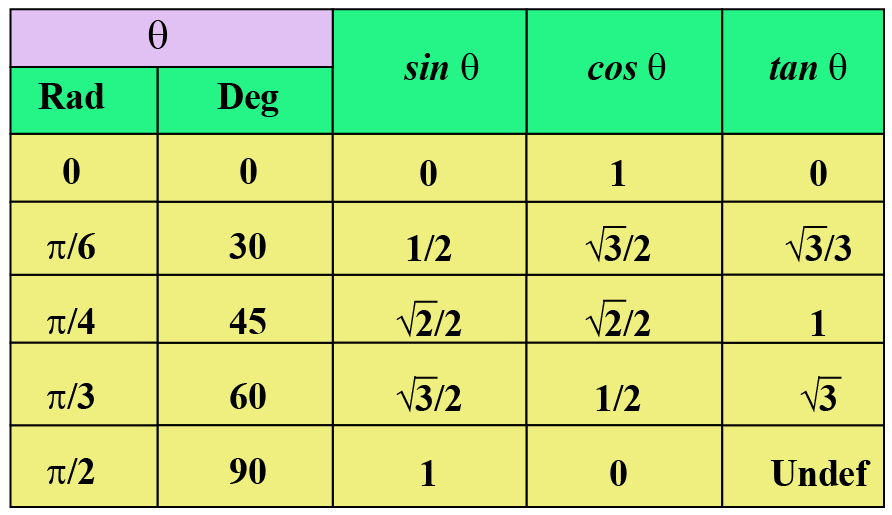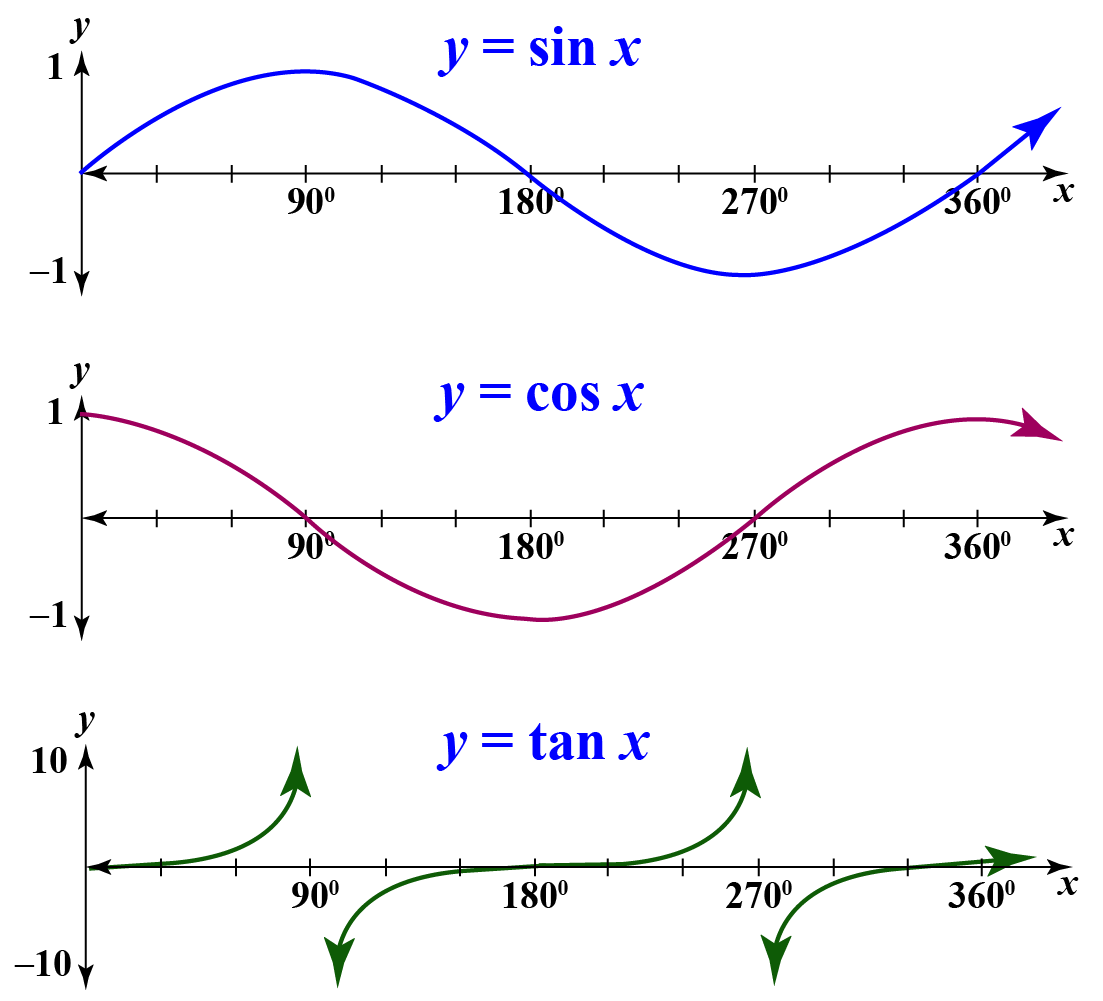In this mini-lesson, we will explore the world of tangent function in math.

You will get to learn about the tangent formula, tangent meaning, range and domain of the tangent function, tan function graph, trigonometric ratios, trig identities, and other interesting facts around the topic.

As we scroll down, try your hand at solving a few interesting practice questions at the end of the page.

## Lesson Plan

 1 What Is a Tangent Function? 2 Important Notes on Tangent Function 3 Solved Examples on Tangent Function 4 Thinking out of the Box! 5 Interactive Questions on Tangent Function

## What Is a Tangent Function?

The tangent function is one of the basic trigonometric functions.

Tangent is defined as the ratio of the opposite side to the adjacent side of a specific angle of a right-angled triangle.

Let's look at the right-angled triangle $$ABC$$ shown below.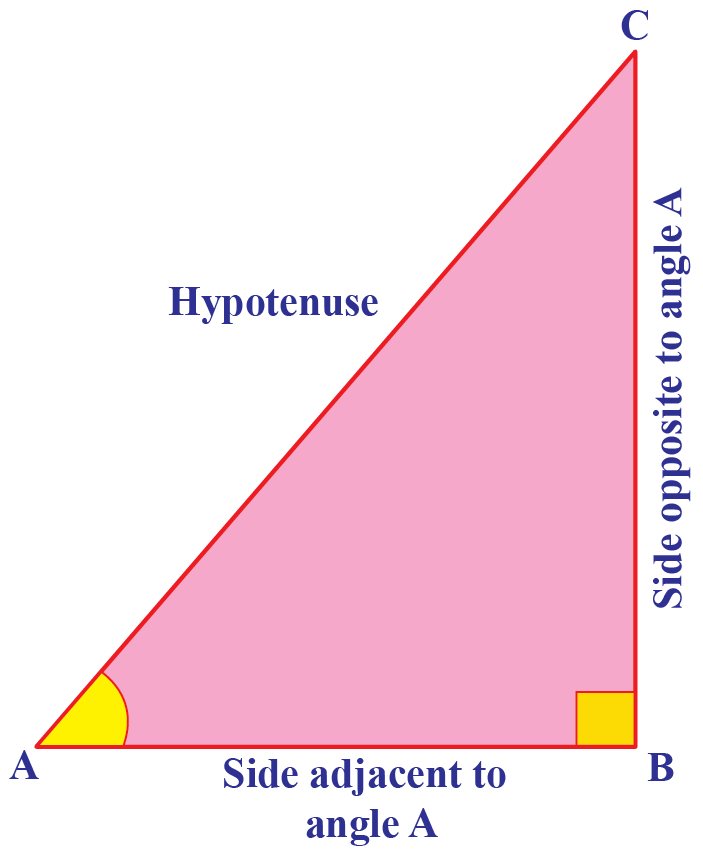$$AC$$ is the hypotenuse.

The base $$AB$$ is the extended portion of $$\angle A$$.

Hence, we call it the "side adjacent to $$\angle A$$".

The perpendicular side $$BC$$ faces $$\angle A$$.

Hence, we call it the "side opposite to $$\angle A$$".

Hence, the tangent formula is:

 $$\tan{A}=\dfrac{\text{Side Opposite to } \angle A}{\text{Side Adjacent to }\angle A}=\dfrac{BC}{AB}$$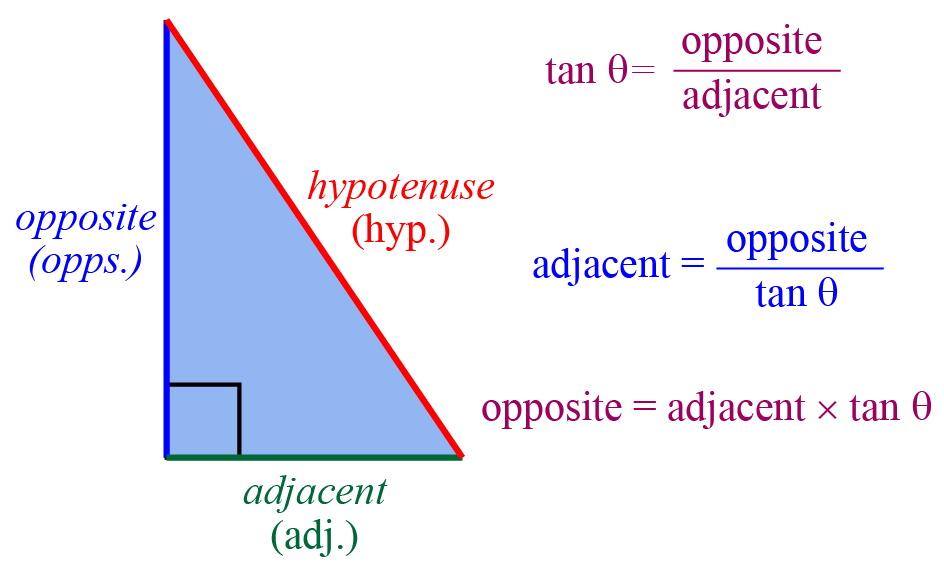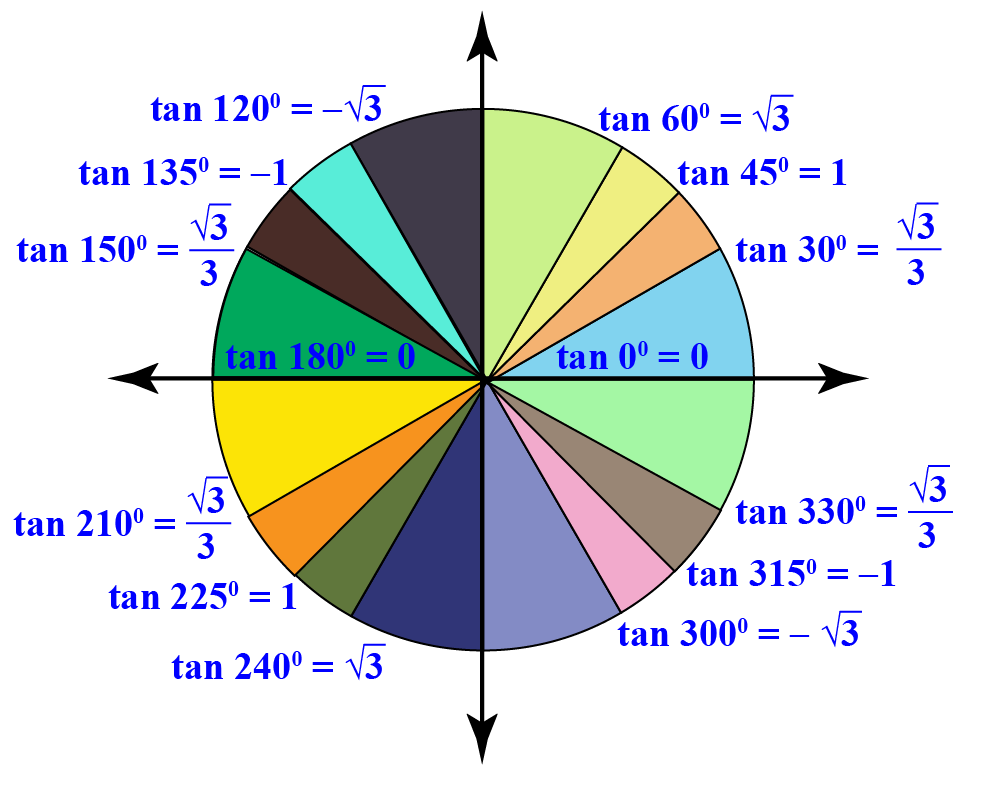### Tangent Using a Unit Circle

Let's understand the tangent meaning using a unit circle.

Look at the unit circle shown below.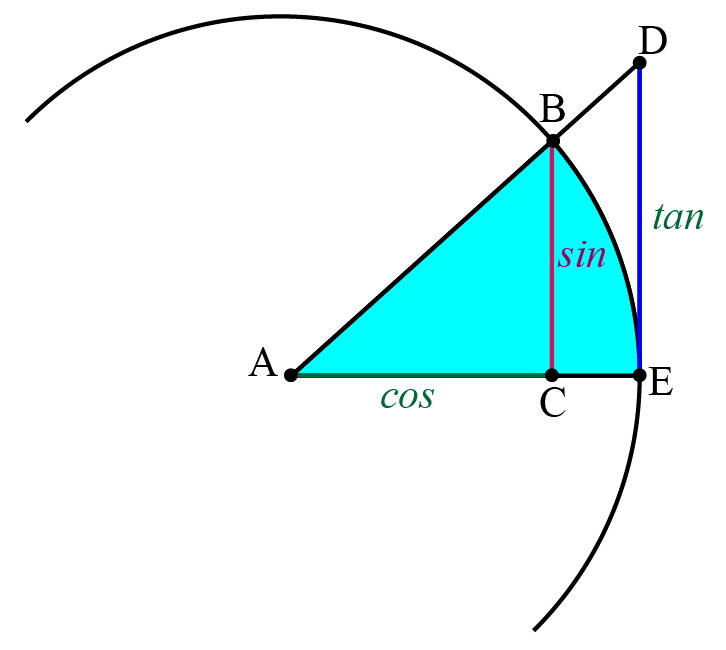The vertical line ED which touches the circle at only one point; at the point of tangency, E.

The triangles $$\Delta ABC$$ and $$\Delta ADE$$ are similar.

This follows that,

\begin{align}\dfrac{ED}{AE}&=\dfrac{CB}{AC}\\ \dfrac{\tan{A}}{1}&=\dfrac{\sin{A}}{\cos{A}}\\ \tan{A}&=\dfrac{\sin{A}}{\cos{A}}\end{align}

## Basic Properties of Tangent Function

Some of the basic properties of tangent function are:

1. The values of $$\tan{x}$$ is undefined where $$\cos{x}=0$$.
2. The tangent function has infinite vertical asymptotes.
3. The tangent function is the ratio of sine function and cosine function, that is, $$\tan{x}=\dfrac{\sin{x}}{\cos{x}}$$.

Though we can find the values of cos, sin, and tan using the calculator, we can also refer to charts that have some standard angles 0o, 30o, 45o, 60o, and 90o.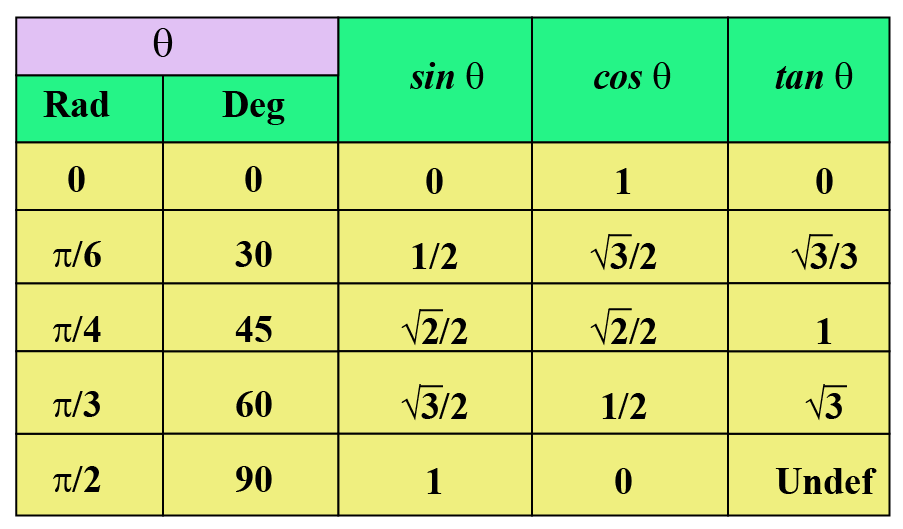### Trigonometric Identities

An equation becomes an identity when it is true for all the variables involved in it.

Now, we will discuss trig identities involving tangent function which are true for all angles involved in it.

 $$1+\tan^{2}{A}=\sec^{2}{A}$$
 $$\tan{(2A)}=\dfrac{2\tan{A}}{1-\tan^{2}{A}}$$
 $$\sin{(2A)}=\dfrac{2\tan{A}}{1+\tan^{2}{A}}$$

## Tan Function Graph

Look at the tan function graph shown below.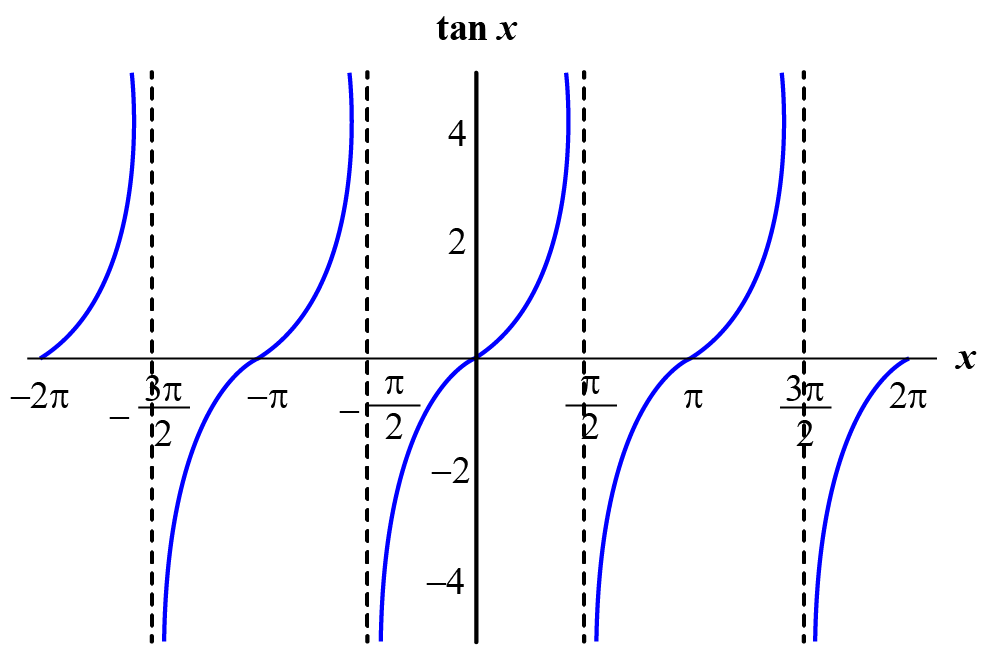Did you notice that the pattern of curves is repeating after an interval of $$\pi$$?

Also, observe that the values of $$\tan{x}$$ increases as $$x$$ increases in $$\left(0,\dfrac{\pi}{2}\right)$$.

The function has vertical asymptotes at $$x=\dfrac{\pi}{2}, \dfrac{3\pi}{2}, -\dfrac{\pi}{2}$$.

Range and Domain of Tangent Function and Other Properties

We make the following observations:

• The $$\tan x$$ functions is not defined for all odd multiples of $$\frac{\pi }{2}$$. This is because the base is 0 for these positions. Thus, the domain of the $$\tan x$$ function is $$\mathbb{R}\ - \left\{ {\left( {2n + 1} \right)\frac{\pi }{2}} \right\}$$, where $$n$$ belongs to the set of integers.

• The range of this function is $$\mathbb{R}$$, as the $$y$$-variation in the graph is from negative infinity to positive infinity.

• The $$\tan x$$ function has a cycle of $$\pi$$, instead of $$2\pi$$.

Domain of the $$\tan x$$ function is $$\mathbb{R}\ - \left\{ {\left( {2n + 1} \right)\frac{\pi }{2}} \right\}$$, where $$n$$ belongs to the set of integers and the range of $$\tan x$$ function is $$\mathbb{R}$$.

The other properties of the tangent function are listed below:

## Solved Examples

 Example 1

Find the tan value of the following triangle for the given angle $$\theta$$.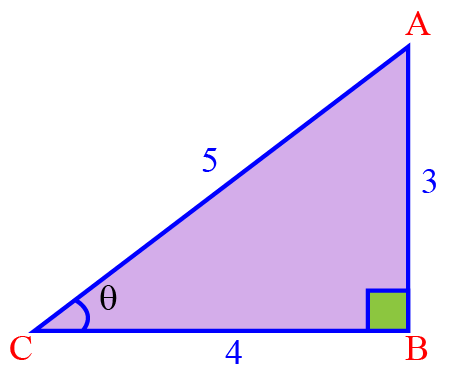Solution

In this triangle, the longest side or the side opposite to the right angle is $$AC$$ and hence, $$AC$$ is the hypotenuse.

The side opposite to $$\theta$$ is $$AB$$ and hence, $$AB$$ is the opposite side.

The side adjacent to $$\theta$$ is $$BC$$ and hence, $$BC$$ is the adjacent side.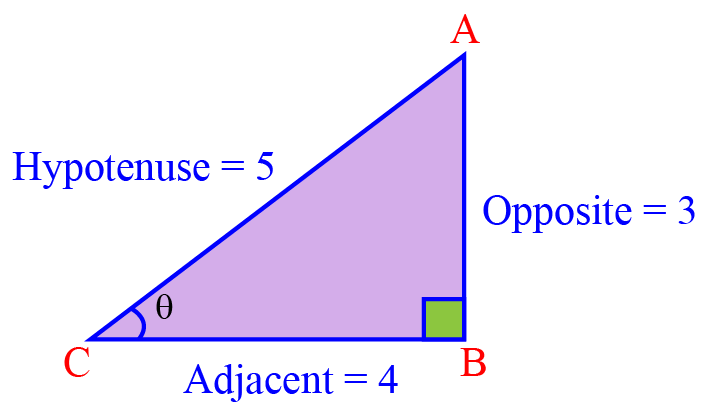Now we will find $$\tan \theta$$ using the tan formula:

\begin{align}\tan\theta &= \dfrac{ \text{Opposite}}{ \text{Adjacent}}=\dfrac{AB}{BC}=\dfrac{3}{4}\\[0.2cm] \end{align}

 $$\therefore$$ $$\tan\theta = \dfrac{3}{4}$$
 Example 2

Find the exact length of the shadow cast by a 15 ft tree when the angle of elevation of the sun is 60º.

Solution

Let us assume that the length of the shadow of the tree is $$x$$ ft.

Using the given information: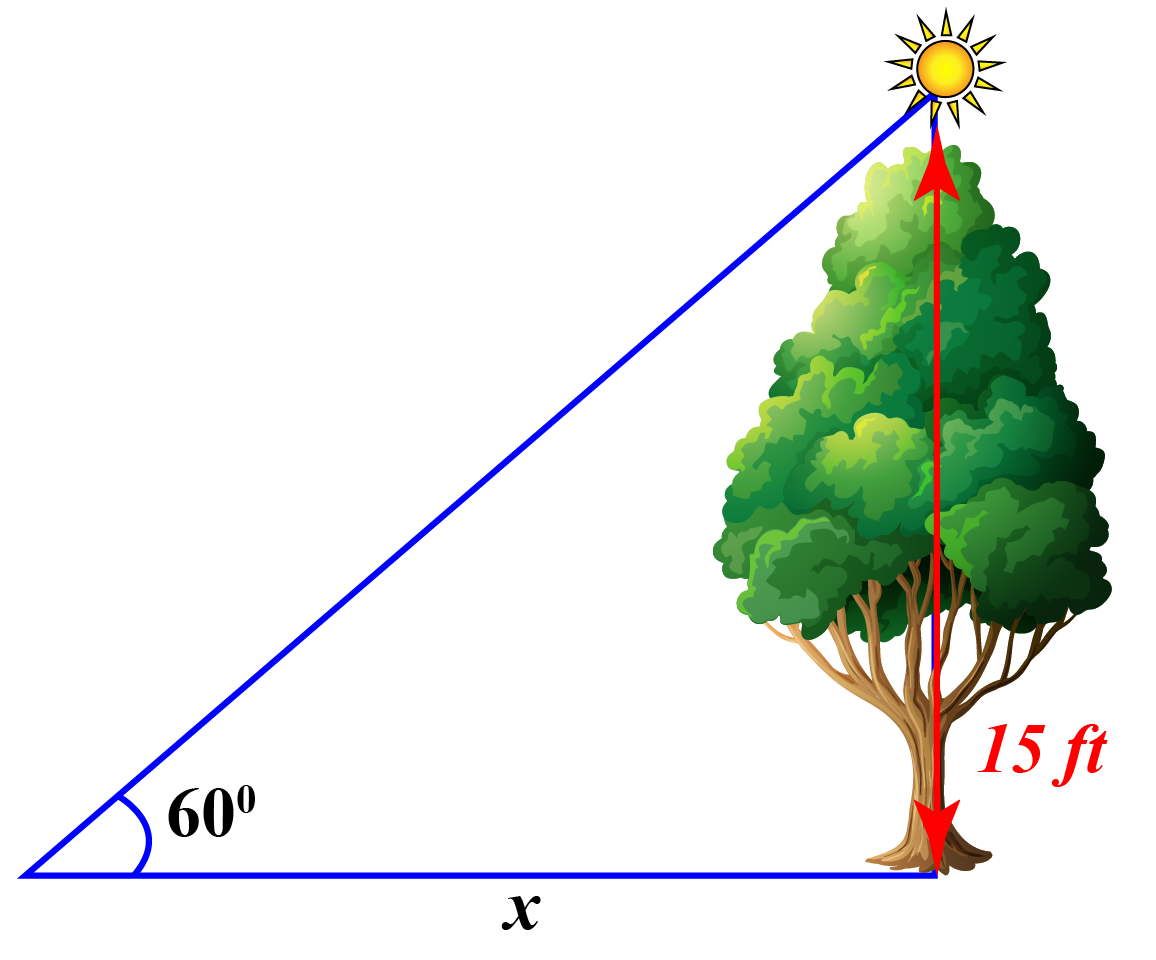Applying tan to the given triangle

\begin{align} \tan 60^\circ &= \dfrac{15}{x}\\[0.2cm] x&= \dfrac{15}{\tan 60^\circ}\\[0.2cm] x &= \dfrac{15}{\sqrt 3} \,\,\, \text{(Using chart)} \\[0.2cm] x&= \dfrac{15 \sqrt 3}{3}\\ &x=5 \sqrt 3 \end{align}

 $$\therefore$$ The length of the shadow of the tree  is $$5 \sqrt 3$$ ft
 Example 3

Jack is standing on the ground and looking at the top of the tower with an angle of elevation of $$60^{\circ}$$.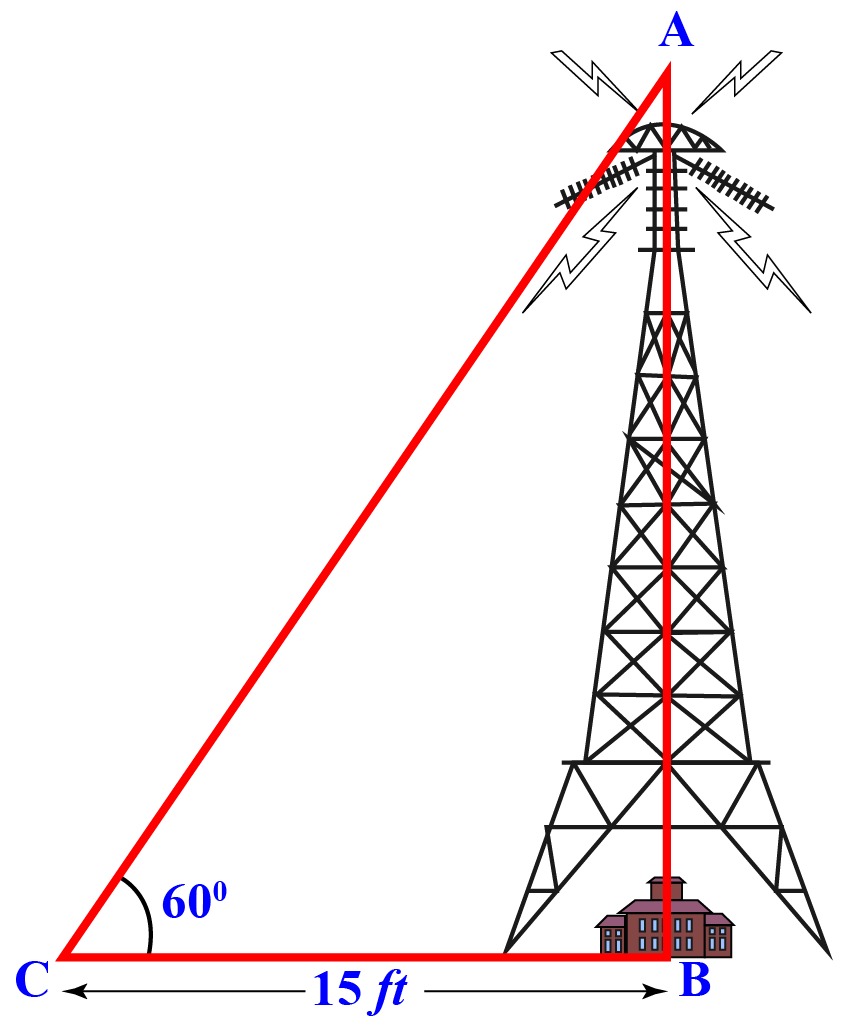If he is standing 15 feet away from the foot of the tower, can you determine the height of the tower?

### Solution

Since the ratio involves the sides $$AB$$ and $$BC$$, we will use the trigonometric ratio $$\tan{60^{\circ}}$$.

\begin{align}\tan{60^{\circ}}&=\dfrac{AB}{BC}\\\sqrt{3}&=\dfrac{AB}{15}\\AB&=15\sqrt{3}\end{align}

 $$\therefore$$ The height of the tower is $$15\sqrt{3}$$ feet.Think Tank
1. Can you determine the nature of the tangent function in 4 quadrants of the XY-plane using graphical representation?
2. Can you draw the graph of $$\cot{x}$$ using the graph of $$\tan{x}$$?

## Interactive Questions

Here are a few activities for you to practice.

## Let's Summarize

We hope you enjoyed learning about the Tangent Function with the examples and practice questions. Now, you will be able to easily solve problems on the tangent function in trigonometry.

At Cuemath, our team of math experts is dedicated to making learning fun for our favorite readers, the students!

Through an interactive and engaging learning-teaching-learning approach, the teachers explore all angles of a topic.

Be it worksheets, online classes, doubt sessions, or any other form of relation, it’s the logical thinking and smart learning approach that we, at Cuemath, believe in.

## FAQs on Tangent Function

### 1. How do you use the word tangent?

The word tangent is used to address a line that touches a circle at a unique point.

### 2. Why is Tan called tangent?

The expanded form of tan is tangent. Tan function is related to the tangent of a unit circle.

### 3. How do you find tangent?

We can find the tangent using the following two ratios:

1. $$\tan{x}=\dfrac{\sin{x}}{\cos{x}}$$
2. $$\tan{\theta}=\dfrac{\text{Side Opposite to } \angle \theta}{\text{Side Adjacent to }\angle \theta}$$

### 4. How do you know if a function is periodic?

If the graph of a function keeps repeating its patterns after a definite interval, we say that the function is periodic in nature.

### 5. How do you find the tangent of a triangle?

We can find the tangent of a triangle using the following two ratios:

1. $$\tan{x}=\dfrac{\sin{x}}{\cos{x}}$$
2. $$\tan{\theta}=\dfrac{\text{Side Opposite to } \angle \theta}{\text{Side Adjacent to }\angle\theta}$$

### 6. What is the tangent rule?

The tangent rule is given by the following two ratios:

1. $$\tan{x}=\dfrac{\sin{x}}{\cos{x}}$$
2. $$\tan{\theta}=\dfrac{\text{Side Opposite to } \angle\theta}{\text{Side Adjacent to }\angle\theta}$$

### 7. What is tangent function in trigonometry?

We define the tangent function or tangent ratio of an angle as the ratio of the length of the opposite side to the length of the adjacent side with respect to a particular angle in a right triangle.

Trigonometry
Trigonometry
Grade 9 | Questions Set 1
Trigonometry
Trigonometry
Grade 10 | Questions Set 1
More Important Topics
Numbers
Algebra
Geometry
Measurement
Money
Data
Trigonometry
Calculus
More Important Topics
Numbers
Algebra
Geometry
Measurement
Money
Data
Trigonometry
Calculus
Learn from the best math teachers and top your exams

• Live one on one classroom and doubt clearing
• Practice worksheets in and after class for conceptual clarity
• Personalized curriculum to keep up with school